# 05.01 Distributions¶

We saw measures about the data, and we saw the assumption of the standard deviation. Why is this standard deviation thing important? Because it describes how the data variates from the mean, and how that moves from the mean becomes apparent if we assume that our data can be described by a Gaussian (normal) distribution.

We import our common suspects.

In :
import numpy as np
import pandas as pd
import matplotlib.pyplot as plt
%matplotlib inline
plt.style.use('seaborn-talk')
from scipy import stats


And since that normal (Gaussian) distribution is so important we shall plot it. The equation of a normal distribution is defined in code in the gaussian procedure below. Then we perform an extensive exercise in plotting.

Note that the arguments for the gaussian procedure may appear strange: we have the mean and both the standard deviation and the variance. For a Gaussian distribution one does not need both, one could - and probably should - calculate the standard deviation form the variance or vice-versa. The three arguments are included for you to play with. In a strange world where $\sigma \neq \sqrt(\sigma^2)$ a normal distribution will not look like a normal distribution. Moreover, the relationship of variance and standard deviation is what makes a normal distribution a normal distribution. One interesting set of arguments to try may be std=1 and var=3, for a graph of a distribution that is not normal.

In :
def gaussian(x, mean=0, std=1, var=1):
return np.exp(-(x-mean)**2/2*var)/(np.sqrt(2*np.pi*var))

arrow = {'facecolor': 'black', 'arrowstyle': '->'}
text = {'fontsize': 14, 'ha': 'center', 'arrowprops': arrow}
fig, ax = plt.subplots(figsize=(16, 9))

x = np.linspace(-4, 4, 128)
y = gaussian(x)
ax.plot(x, y, color='navy')
x = np.linspace(-2, 2, 128)
y = gaussian(x)
ax.fill_between(x, y, alpha=0.2, color='seagreen')
ax.annotate('95.45%', xy=(-1.5, 0.05), xytext=(-3, 0.2), **text)

x = np.linspace(-1, 1, 128)
y = gaussian(x)
ax.fill_between(x, y, alpha=0.5, color='crimson')
ax.annotate('68.27%', xy=(0.5, 0.2), xytext=(1.5, 0.35), **text)

x = np.array([-1, 1])
y = gaussian(x)
ax.scatter(x, y, s=100, color='navy')
ax.annotate('inflection point', xy=(x+0.07, y+0.001), xytext=(3, 0.27), **text)

def plot_std(ax, xy_left, xy_right):
arrow['arrowstyle'] = '<->'
ax.annotate('', xy=xy_left, xytext=xy_right, **text)
xcentre = xy_left + (xy_right - xy_left)/2
ax.text(xcentre, xy_left + 0.01, 'std', ha='center', fontsize=14)
arrow['arrowstyle'] = '->'

plot_std(ax, (-2, 0.01), (-1, 0.01))
plot_std(ax, (-1, 0.01), (0, 0.01))
plot_std(ax, (0, 0.01), (1, 0.01))
plot_std(ax, (1, 0.01), (2, 0.01))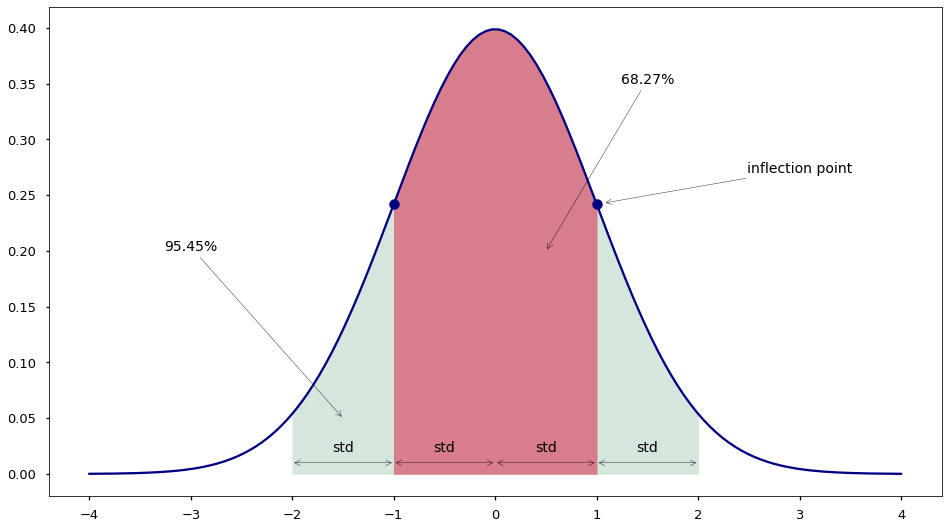The areas below the curve and the inflection points vary according how the Gaussian changes. But the relations always stay true:

• The standard deviation is the horizontal distance between the mean and the inflection point.
• The area within two standard deviations around the mean contains the majority of data.
• The area within four standard deviations contains roughly $95\%$ of the data.

But all that assumes that our data is modeled by a Gaussian distribution. We keep repeating this, and it is time to see things that are not modeled by a normal distribution.

The Gaussian distribution above is a continuous distribution but there are as well discrete distributions. The discrete equivalent to the Gaussian distribution is the Binomial distribution, which is pretty much the histogram of a Gaussian.

Yet, other distribution exist, for example the Poisson discrete distribution models occurrence of an event during a time period. It models the probability of an event occurring a number of times. Here the vertical axis is the probability of number of occurrences $x$ ($1$ to $64$) on the horizontal axis. The different graphs represent different variances of the event occurrences, here variances of $1$, $5$, $10$, $30$ and $50$. Note that the time period is not defined by the distribution, and is required to be interpreted given a problem.

In :
fig, ax = plt.subplots(figsize=(16, 9))

x = np.arange(1, 64)
for gamma in [1, 5, 10, 30, 50]:
y = stats.poisson.pmf(x, gamma)
label = '$\gamma = %s$' % gamma
ax.plot(x, y, '.', label=label)
ax.legend();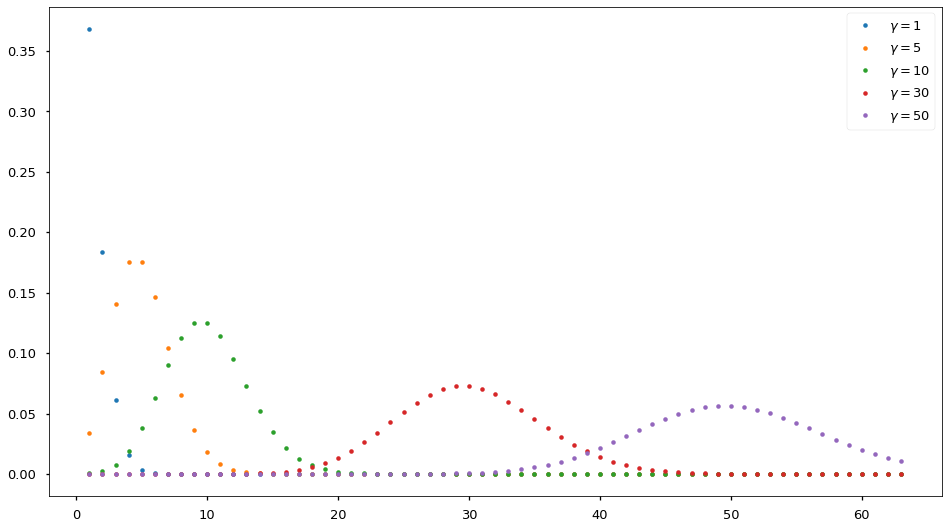And the continuous version of the event based distribution is the Weibull distribution. Well, to be fair the continuous version of the Poisson distribution is the exponential distribution but the Weibull distribution is an extension to the exponential distribution.

The Weibull distribution also models the occurrence of an event in time. Yet, Weibull is often used to get a continuous value, e.g. the occurrence of half an event. The distribution was developed to model material failures, where one wishes to know when an almost failure will occur.

The distribution has an extra parameter (here $k$) which models for the change in the chance of a failure. The biggest the $k$ the faster a failure may happen when time passes.da-weibull.svg
In :
def weibull(x, gamma, k):
return (k/gamma) * (x/gamma)**(k-1) * np.exp(-(x/gamma)**k)

fig, ax = plt.subplots(figsize=(16, 9))
x = np.linspace(0, 4, 256)
for k in [1, 1.5, 3, 5, 10]:
y = weibull(x, 1, k)
label = 'k = %s' % k
ax.plot(x, y, label=label)
ax.legend();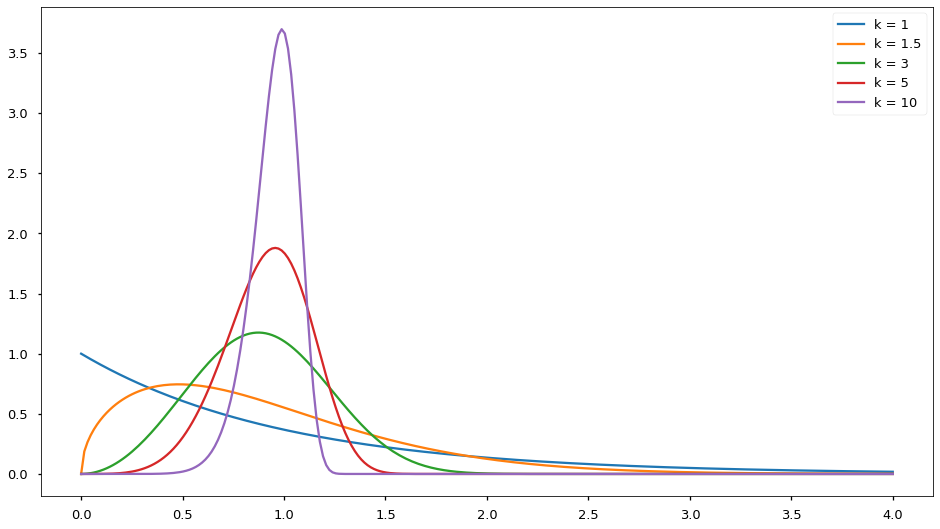Therefore the data can be modeled by quite a lot of different distributions, right? Yes. Yet in the vast majority of cases a Gaussian distribution turns out to be the best model. This is because the sum of several continuous distributions can be approximated as a Gaussian distribution. In other words, when distinct processes influence the data we measure, it is likely that we will see a Gaussian distribution. Since each process affects the measures in a way that may result in some distribution, a group of distinct processes will produce a sum of distinct distributions, which tend towards a Gaussian.

That works for continuous distributions, the sum of discrete distributions vary, yet often can be approximated with the Uniform distribution.

Let's try this out by summing together several Weibull distributions. We plot here the evolution (cumulative sum) of adding more and more Weibull distributions.

In :
x = np.linspace(0, 4, 256)
y = []
fig, ax = plt.subplots(figsize=(16, 9))
ks = [1, 12, 23, 34, 45, 56, 67, 78, 89, 100]
for k in ks:
y.append(weibull(x, 1, k))
s = np.cumsum(np.array(y), axis=0)
for k, i in zip(ks, s):
label = '$k = %s$' % str(k)
ax.plot(x, i, label=label)
ax.legend();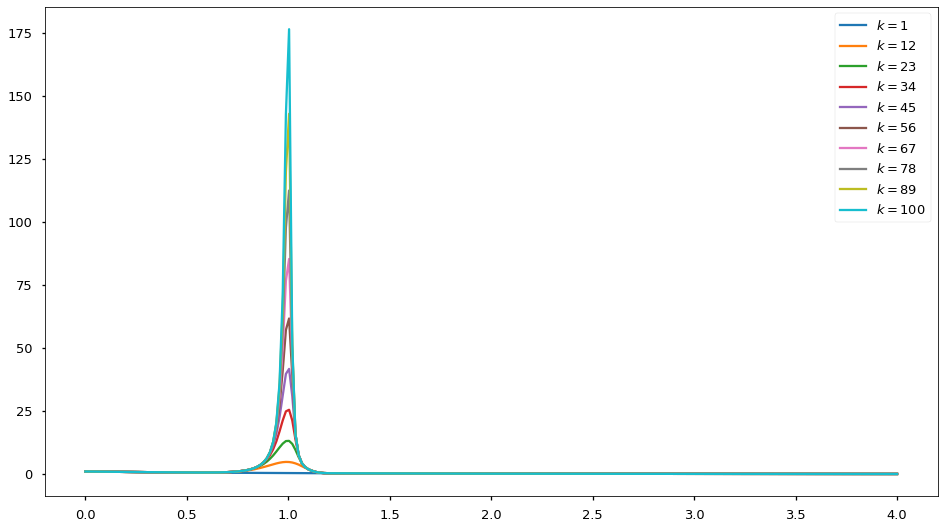The above is often called the Central Limit Theorem in statistics. It works in many problems allowing us to assume the normal distribution of data, and only worry about not normally distributed data when we attempt statistics based on a Gaussian distribution and they fail. Most of the time we will be working with data that is generated by a complex process, i.e. by a combination of several processes. Therefore the Gaussian assumption and the use of standard deviation can be justified.

On the other hand the Central Limit Theorem does not allow us to assume that every problem will be well modeled by a Gaussian distribution. If we change the $k$ values we used for our Weibull distributions above we can easily make a problem for which the Central Limit Theorem doe not work.

In :
x = np.linspace(.01, 4, 256)
y = []
fig, ax = plt.subplots(figsize=(16, 9))
ks = [.1, .2, .3, .4, .5, 9, 10, 12, 13]
for k in ks:
y.append(weibull(x, 1, k))
s = np.cumsum(np.array(y), axis=0)
for k, i in zip(ks, s):
label = '$k = %s$' % str(k)
ax.plot(x, i, label=label)
ax.legend();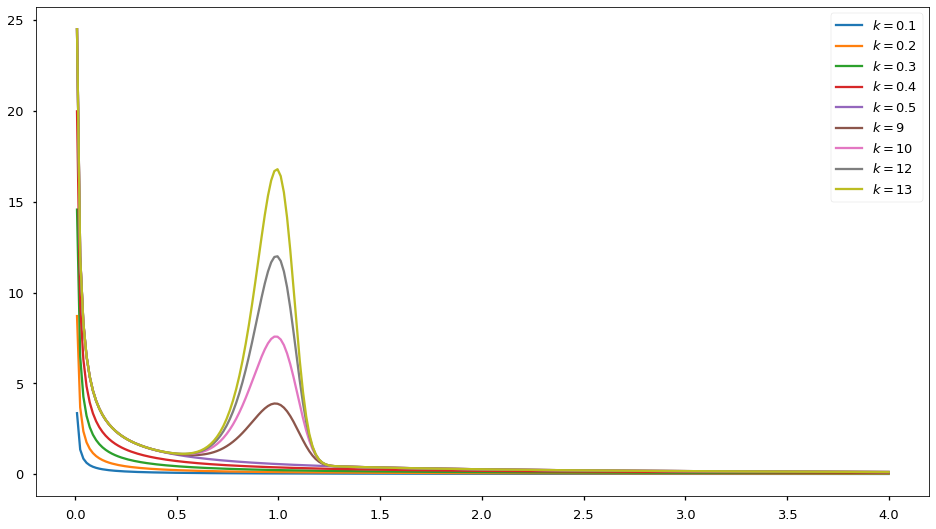Here we see a multivariate (also called a multi-modal) distribution. Despite the fact we accumulated several processes we did not end up with a Gaussian-like distribution. In complex problems this may happen, and we need to be aware of this possibility. Moreover, there is no known single distribution to model this specific combination of processes. Of course, we can model the scenario with several Weibull distributions one fore each process we graph. In real data we do not have access to the information of how many different process and their nature is not available to us. In real world data finding out the processes generating it is our task in the first place.

Most known statistical distributions are univariate (have a single peak), and hence obey the Central Limit Theorem. In this specific case the two peaks happen because we used values of $k$ below $1$, which mean a decreasing chance of failure over time; and values of $k$ above $1$ which mean an increasing chance over time of failure. The difference in meaning causes an effect often called a bifurcation.

The take away message is that it is okay to start out with the assumption that our data is reasonably close to a normal distribution. But it is also important to keep at the back of one's mind the fact that if things go wrong we may be working with a distribution of data that is not normal in any way. Life then becomes complicated. Yet there are methods to work with non-normal data, and knowing that we do not have a normal distribution is better than attempting to square every circle just because working with circles is hard.